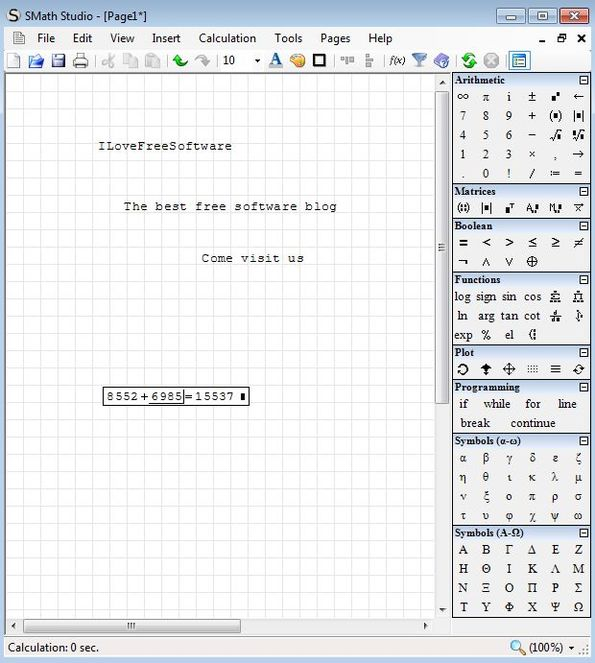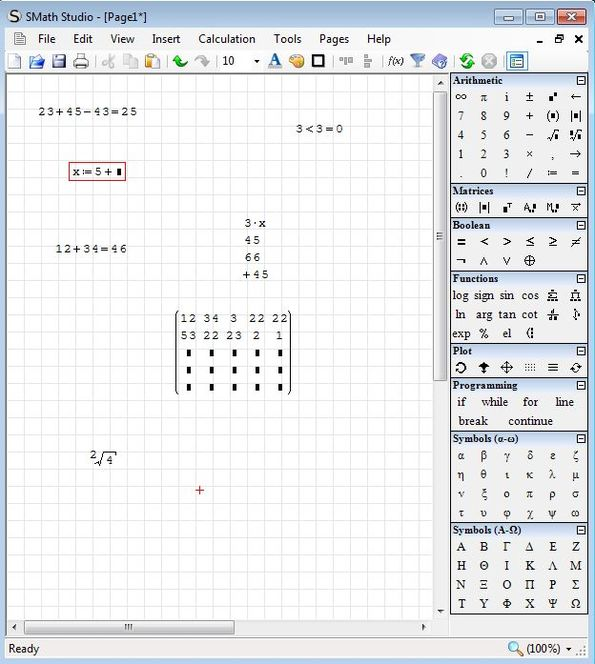Editor Ratings:
User Ratings:
[Total: 0 Average: 0]

SMath Studio is a free software that lets you create and solve advanced math problems with the help of several pre-given tools. It’s like an advanced maths calculator, which can be used to perform advanced mathematical calculations. You can use it for arithmetic, matrices, boolean logic, trigonometric functions, plotting, and even setting up programing problems. It comes with a paper-like working area, where you can setup your problems, and it also supports graphing. Created math problems, their solutions, and generated graphs can be printed or saved in various different formats, including Mathcad.

In the image down below, you can see how the paper-like interface looks like. Entire working area in the central region looks like a math workbook. The right side holds a toolbar that you can use for setting up the equations. Symbols that you can add to the workbook are divided into categories.Apart from the workbook area and the right sidebar, there’s also a standard menu/quick access toolbar combo on the top left corner that you can use.

## Key Features of SMath Studio are:

• Free and simple to use: it does require you to know math of course.
• Supports arithmetic, boolean logic, matrices, trig, function, plotting.
• Can be used in order to create graphs of mathematical functions.
• Supports export of graphs as either PNG, BMP, GIF, JPEG, EXE, SM, SMZ.
• Compatible with the XMCD file format, used by Mathcad.
• Printing: you can print your work directly from inside the application.
• Cross platform: works on both Linux and Windows, Linux requires Mono.

Even though this free advanced math calculator is easy to use, you still need to have knowledge of advanced maths to use it to its full potential. Help tutorials (on download page, link given at the end) are also available that you can use if you stuck anywhere in between, while using SMath Studio.

## Setting Up and Solving Advanced Math Problems with SMath Studio:

To start setting up a math problem, the only thing you need to do is to open up SMath Studio and start defining the problem using the tools available in the side toolbar.Entire workbook can be used, you just need to left click anywhere in order to be able to type in math problems in this advanced math calculator. After entering the problem, just put an “Equal to” and SMath will immediately show the resultant values.

We only sticked to very simple things like basic arithmetics. For something more advanced, you will have to look up the tutorials on the download page (link at the end) to get more familiar with how those things are done.

When you’re done setting up your math problems and would like to see them printed, select File >> Print option. Those who would like to save them as image, select File >> Save As..

## Conclusion

SMath has a lot to offer for a relatively small application (it’s just around 2MB in size). If you’re a teacher and require to solve advanced mathematical problems or setting up question papers for students, then this is the tool that you need to use. It comes with a very large selection. Give this free mathematical utility a try and see how it goes. Download for free and let us know what you think about it.